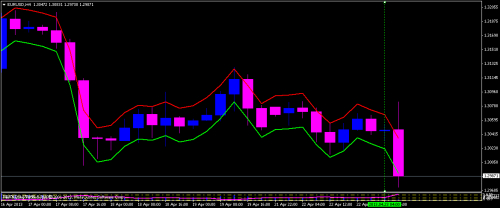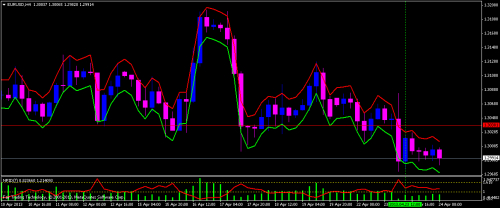In my article Prices, Material Point ... Laws in Common (part 2), I presented a summary of strengths indicators and derivatives thereof, result of a work which aims to build a relevant trading system based on a simulation approach already explained in my articles (Price Movement, Signs of Indicators, Gaps to Reduce)

In this article I presented a version of indicators linked to the price on chart and forms a sort of envelope that identifies the movement area of price. This indicator gives two values simultaneously at current prices, which are obtained by addition (resp. subtraction) of a calculated value based on the buying strength (resp sales strength) and the pivot price value of a unit time (bar).

Idea of ​​calculation

Over an interval of time that the two waves meet a growing and second decreasing , I have noted values ​​buying and sales strength’s values ( tops and bottoms) (see chart)Adjustment exponential analysis establishes a relationship between the buyers and sellers strength.

Relation :

correlation coefficient is very close to value .

Using these relations in the code indicator ‘linked’ to the price we got an envelope that identifies the price and predicts the time of change of direction.Introduction of the Indicator

The red curve represents the boundary around which the current strength of the buyers ended and will be completed, values ​​are obtained by the following formula:

NPIdDIVExtMapBuffer[i]=(pivot+((2.593*MathExp(-0.76*(NPIDIVExtMapBuffer[i])))/300)*pivot)-0.002;

The green curve represents limit curve around which the current strength of the buyers will be completed, values ​​are obtained by the following formula:

NPIDIVExtMapBuffer[i]=(pivot-(2.795*MathExp(-0.081*(NPIuExtMapBuffer[i]))/300)*pivot)+0.009;

By looking at the chart it is clear that when the price touches the lower value the sales force ended and reaches its limit then new direction is expected just with the closing of the bar touched the green curve and opening of a new bar above this curve.

Even when the of the price touches the upper value (red curve) the buying power is ended and reaches its limit then new direction is expected just with the closing of the bar touched the red line and the opening of a new bar below this curve.

ApplicationHonestly this application is the first right after the compilation of the indicator, the goal is to confirm the effectiveness of what we have discussed above and make sure that the indicator actually identifies the award as it shows the chart.
The sample application shows that the price strictly respects (Bands) which can give very good results.

Translate to English Show original